# Reverse Monte Carlo

Reverse Monte Carlo (RMC)  is a variation of the standard Metropolis Monte Carlo method. It is used to produce a 3 dimensional atomic model that fits a set of measurements (neutron diffraction, X-ray-diffraction, EXAFS etc.). In addition to measured data a number of constraints based on prior knowledge of the system (for example, chemical bonding etc.) can be applied. Some examples are:

• Closest approach between atoms (hard sphere potential)
• Coordination numbers.
• Angles in triplets of atoms.

The 3 dimensional structure that is produced by reverse Monte Carlo is not unique; it is a model consistent with the data and constraints provided.

The algorithm for reverse Monte Carlo can be written as follows:

1. Start with a configuration of atoms with periodic boundary conditions. This can be a random or a crystalline configuration from a different simulation or model.
2. Calculate the total radial distribution function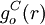$g_o^C(r)$ for this old configuration (C=Calculated, o=Old).
3. Transform to the total structure factor: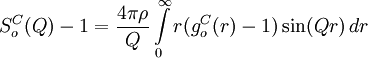$S_o^C (Q)-1=\frac{4\pi\rho}{Q}\int\limits_{0}^{\infty} r(g_o^C(r)-1)\sin(Qr)\, dr$
where Q is the momentum transfer and$\rho$ the number density.
4. Calculate the difference between the measured structure factor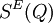$S^E(Q)$ (E=Experimental) and the one calculated from the configuration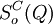$S_o^C(Q)$: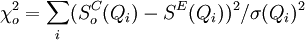$\chi_o^2=\sum_i(S_o^C(Q_i)-S^E(Q_i))^2/\sigma(Q_i)^2$
this sum is taken over all experimental points$\sigma$ is the experimental error.
5. Select and move one atom at random and calculate the new (n=New) distribution function, structure factor and: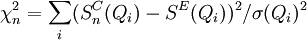$\chi_n^2=\sum_i(S_n^C(Q_i)-S^E(Q_i))^2/\sigma(Q_i)^2$
6. If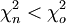$\chi_n^2<\chi_o^2$ accept the move and let the new configuration become the old. If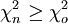$\chi_n^2 \geq \chi_o^2$ then the move is accepted with probability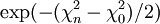$\exp(-(\chi_n^2-\chi_0^2)/2)$ otherwise it is rejected.
7. repeat from step 5.

When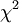$\chi^2$ have reached an equilibrium the configuration is saved and can be analysed.